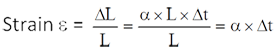# Technical Questions asked in Reliance Industries

1. A steel rod of length L and diameter D, fixed at both ends, is uniformly heated to a temperature rise of ΔT. The Young's modulus is E and the coefficient of linear expansion is a. The thermal stress in the rod is(Mechnical Engg.)
1. Zero
2. αΔT
3. EαΔT
4. EαΔTL
Due to Af rise in temperature of steel rod of length L, increase in length
ΔL = α × L × Δt
Since, both ends are fixed, thermal stress will be produced in the steel rod.∴ Thermal stress σ = E × ε = E × α × Δt
2. In terms of Poisson's ratio (v), the ratio of Young's modulus (E) to shear modulus (G) of elastic materials is(Mechnical Engg.)
1. 2(1+v)
2. 2(1-v)
3. 1/2 (1+v)
4. 1/2 (1-v)
We know that Young's modulus (E) is connected to shear modulus (G) as
E = 2G (1 + v)
where v = Poisson's ratio.
∴ Rigid ratio E/G = 2(1+v)
1. The total area under the stress-strain curve of a mild steel specimen tested upto failure under tension is a measure of (Mechnical Engg.)
1. Ductility
2. Ultimate strength
3. Stiffness
4. Toughness
The total area under tensile stress-strain curve of a specimen upto failure is a measure of toughness of the material.
2. The depth of flow in an alluvial channel is 1.5 m. If critical velocity ratio is 1.1 and Manning's n is 0.018,the critical velocity of the channel as per Kennedy's method is (Civil Engg.)
1. 0.713m/s
2. 0.784 m/s
3. 0.879 m/s
4. 1.108m/s
y = 1.5m,n=0.018
Critical velocity as per Kennedy's equation
v = 0.55 m/s064
= 6.55×1.1×(1.5)064
= 0.784 m/s
3. A sprinkler irrigation system is suitable when
1. the land gradient is steep and the soil is easily erodible
2. the soil is having low permeability
3. the water table is low
4. the crops to be grown have deep roots